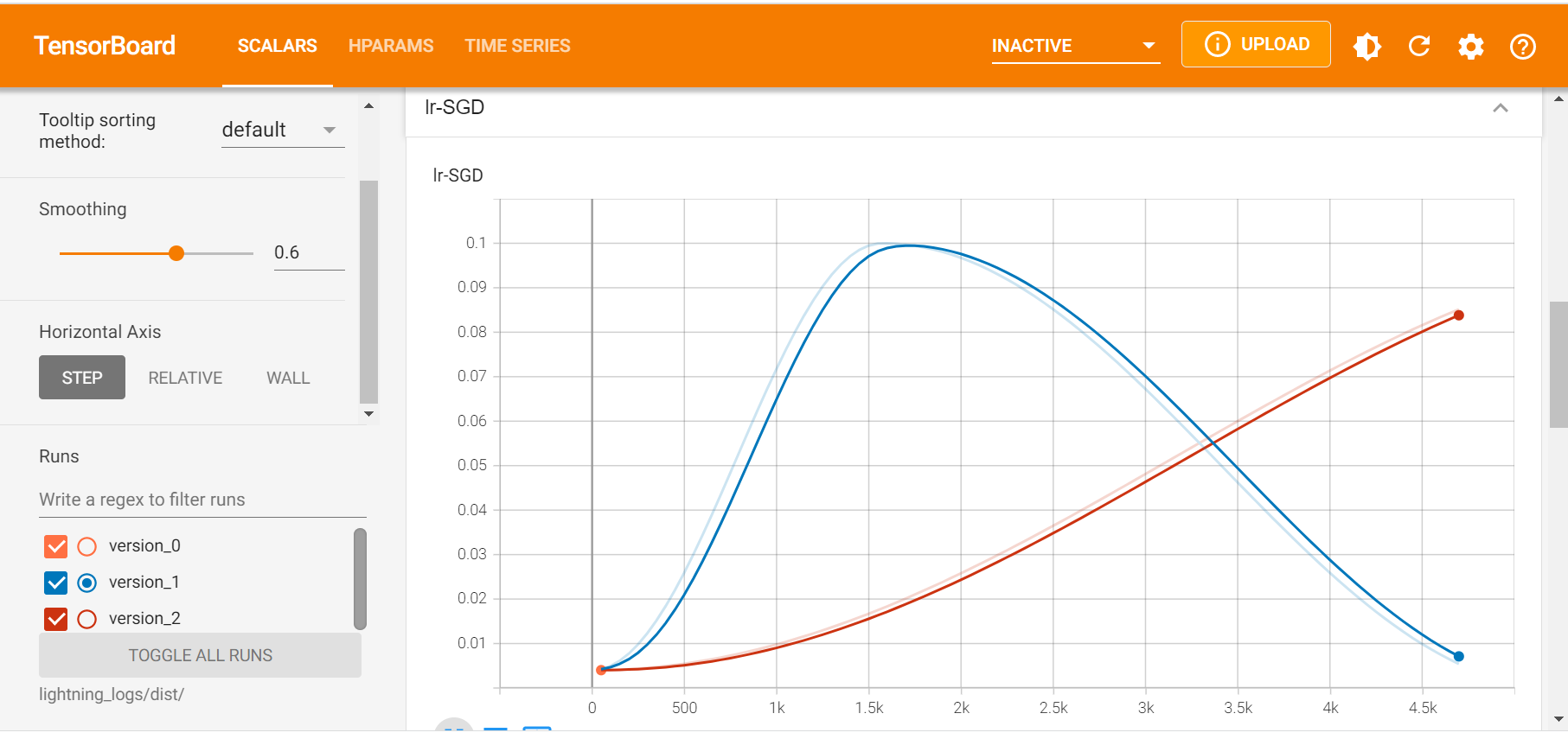# BigDL-Nano PyTorch Trainer Quickstart#

In this guide we will describe how to scale out PyTorch programs using Nano Trainer in 5 simple steps

## Step 0: Prepare Environment#

We recommend using conda to prepare the environment. Please refer to the install guide for more details.

```conda create py37 python==3.7.10 setuptools==58.0.4
conda activate py37
# nightly bulit version
# set env variables for your conda environment
source bigdl-nano-init
pip install lightning-bolts
```

## Step 1: Import BigDL-Nano#

The PyTorch Trainer (`bigdl.nano.pytorch.Trainer`) is the place where we integrate most optimizations. It extends PyTorch Lightning’s Trainer and has a few more parameters and methods specific to BigDL-Nano. The Trainer can be directly used to train a `LightningModule`.

```from bigdl.nano.pytorch import Trainer
```

Computer Vision task often needs a data processing pipeline that sometimes constitutes a non-trivial part of the whole training pipeline. Leveraging OpenCV and libjpeg-turbo, BigDL-Nano can accelerate computer vision data pipelines by providing a drop-in replacement of torch_vision’s `datasets` and `transforms`.

```from bigdl.nano.pytorch.vision import transforms
```

## Step 2: Load the Data#

You can define the datamodule using standard LightningDataModule

```from pl_bolts.datamodules import CIFAR10DataModule
train_transforms = transforms.Compose(
[
transforms.RandomCrop(32, 4),
transforms.RandomHorizontalFlip(),
transforms.ToTensor()
]
)
cifar10_dm = CIFAR10DataModule(
data_dir=os.environ.get('DATA_PATH', '.'),
batch_size=64,
train_transforms=train_transforms
)
return cifar10_dm
```

## Step 3: Define the Model#

You may define your model, loss and optimizer in the same way as in any standard PyTorch Lightning program.

```import torch
import torch.nn as nn
import torch.nn.functional as F
import torchvision
from pytorch_lightning import LightningModule

def create_model():
model = torchvision.models.resnet18(pretrained=False, num_classes=10)
model.conv1 = nn.Conv2d(3, 64, kernel_size=(3, 3), stride=(1, 1), padding=(1, 1), bias=False)
model.maxpool = nn.Identity()
return model

class LitResnet(LightningModule):
def __init__(self, learning_rate=0.05, num_processes=1):
super().__init__()

self.save_hyperparameters('learning_rate', 'num_processes')
self.model = create_model()

def forward(self, x):
out = self.model(x)
return F.log_softmax(out, dim=1)

def training_step(self, batch, batch_idx):
x, y = batch
logits = self(x)
loss = F.nll_loss(logits, y)
self.log("train_loss", loss)
return loss

def configure_optimizers(self):
optimizer = torch.optim.SGD(
self.parameters(),
lr=self.hparams.learning_rate,
momentum=0.9,
weight_decay=5e-4,
)
steps_per_epoch = 45000 // BATCH_SIZE // self.hparams.num_processes
scheduler_dict = {
"scheduler": OneCycleLR(
optimizer,
0.1,
epochs=self.trainer.max_epochs,
steps_per_epoch=steps_per_epoch,
),
"interval": "step",
}
return {"optimizer": optimizer, "lr_scheduler": scheduler_dict}
```

For regular PyTorch modules, we also provide a “compile” method, that takes in a PyTorch module, an optimizer, and other PyTorch objects and “compiles” them into a `LightningModule`. You can find more information from here

## Step 4: Fit with Nano PyTorch Trainer#

```model = LitResnet(learning_rate=0.05)
single_trainer = Trainer(max_epochs=30)
single_trainer.fit(model, datamodule=cifar10_dm)
```

At this stage, you may already experience some speedup due to the optimized environment variables set by source bigdl-nano-init. Besides, you can also enable optimizations delivered by BigDL-Nano by setting a paramter or calling a method to accelerate PyTorch or PyTorch Lightning application on training workloads.

### Increase the number of processes in distributed training to accelerate training.#

```model = LitResnet(learning_rate=0.1, num_processes=4)
single_trainer = Trainer(max_epochs=30, num_processes=4)
single_trainer.fit(model, datamodule=cifar10_dm)
```
• Note: Here we use linear scaling rule to imporve the performance of model on distributed training. You can find more useful tricks on distributed computing from the paper published by Facebook AI research(FAIR).

• Note: If you’re using a step related `lr_scheduler`, the value of lr_scheduler’s pre_epoch_steps need to be modified accordingly, or the learning rate may not changes as expected. The change in learning_rate is shown in the following figure, where the blue line is the excepted change and the red one is the case when the pre_epoch_steps remain unchanged.